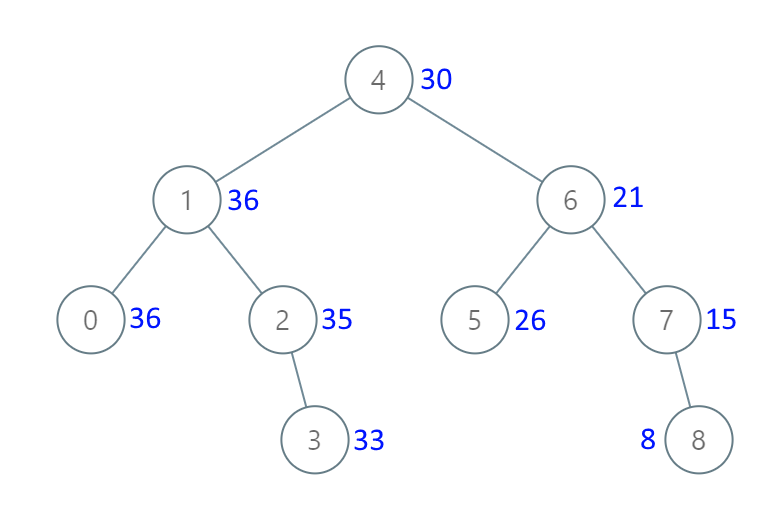# LeetCode: 1038. Binary Search Tree to Greater Sum Tree

## 题目¶

Given the root of a binary search tree with distinct values, modify it so that every node has a new value equal to the sum of the values of the original tree that are greater than or equal to node.val.

As a reminder, a binary search tree is a tree that satisfies these constraints:

The left subtree of a node contains only nodes with keys less than the node's key. The right subtree of a node contains only nodes with keys greater than the node's key. Both the left and right subtrees must also be binary search trees.

Example 1:```Input: [4,1,6,0,2,5,7,null,null,null,3,null,null,null,8]
Output: [30,36,21,36,35,26,15,null,null,null,33,null,null,null,8]
```

Constraints:

1. The number of nodes in the tree is between 1 and 100.
2. Each node will have value between 0 and 100.
3. The given tree is a binary search tree.

Note: This question is the same as 538: https://leetcode.com/problems/convert-bst-to-greater-tree/

## 解法¶

### 从大到小遍历 BST，遍历过程中累加 value¶

```def order(root):
if root is None:
return
order(root.right)
# print(root)
order(root.left)
```

```# Definition for a binary tree node.
# class TreeNode:
#     def __init__(self, val=0, left=None, right=None):
#         self.val = val
#         self.left = left
#         self.right = right
class Solution:
def __init__(self):
self.sum_val = 0

def bstToGst(self, root):
if root is None:
return root

self.bstToGst(root.right)

root.val = root.val + self.sum_val
self.sum_val = root.val

self.bstToGst(root.left)

return root
```